# RRB PO Prelims Quantitative Aptitude Quiz – 15

## RRB PO Prelims Quantitative Aptitude Quiz

Quantitative Aptitude is the most significant part of almost all competitive exams. Candidates appear to be having difficulty with Quantitative Aptitude Questions, so they need to practice more. Even if the portion is difficult, if you practice enough questions and learn the basic concepts, you can surely do well. In this article, we have come up with the RRB PO Quantitative Aptitude Quiz to enhance your preparation. This RRB PO Quantitative Aptitude Quiz will assist you to cope with the tough competition. This RRB PO Quantitative Aptitude Quiz is available to you at no cost. Through this RRB PO Quantitative Aptitude Quiz, you will learn formulas and short tricks to solve the Quantitative Aptitude Questions. Candidates must solve this RRB PO Quantitative Aptitude Quiz to improve their exam preparation.

1. Find the total profit on selling 18 articles whose cost price is 25% less than the mark price and a 15% discount is given on each article while selling price of each article is 34.
(a) 90
(b) 72
(c) 54
(d) 108
(e) 812. Ratio between perimeter of a rectangle and length of that rectangle is 20 : 7. If area of rectangle is 336 cm², then find the volume of cylinder, whose radius is half of the length of rectangle and height is equal to breadth of rectangle ?
(a) 7684 cm²
(b) 7988 cm²
(c) 7866 cm²
(d) 7464 cm²
(e) 7392 cm²3. There are sixteen students in a class, in which 11 boys and 5 girls. If three students selecting randomly for annual debate competition, find probability that at least two girls are selected?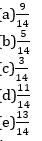4. Train Q of 180 meters length crosses Train P of 120 meters length which is running in same direction in 60 sec. If ratio between speed of train P and train Q is 5 : 6, then find the time taken by both trains to cross each other, when they running in opposite direction?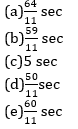5. A can do a piece of work in 48 days and B can do the same work in 64 days, while C take as much time as A and B take together. If they start work alternatively, starting by\ C, followed by B and then A respectively, then find how many days will required to complete the work?
(a) 45 days
(b) 47 days
(c) 39 days
(d) 41 days
(e) 31 days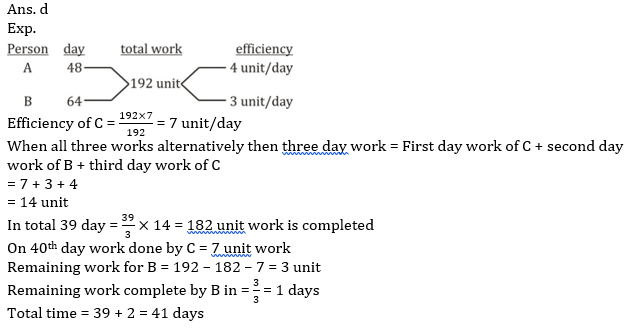Directions (6-10): In the following questions, two equations numbered I and II are given. You have to solve both the equations and give answers among the following options:

6. I. 6x² – 40x + 66 = 0
II. 14y² – 58y + 60 = 0
(a) if x > y
(b) if x ≥ y
(c) if x < y
(d) if x ≤ y
(e) if x = y or the relation between x and y can’t be established.7. I. 22x² – 70x + 12 = 0
II. y² – 9y + 20 = 0
(a) if x > y
(b) if x ≥ y
(c) if x < y
(d) if x ≤ y
(e) if x = y or the relation between x and y can’t be established.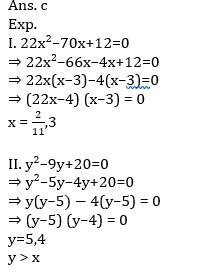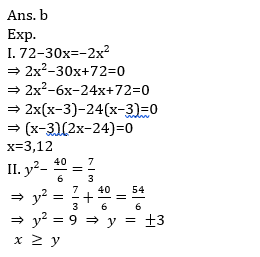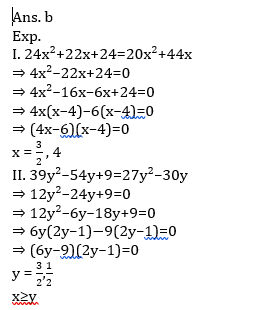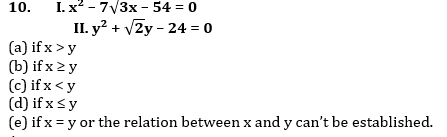###Click to Buy Bank MahaCombo PackageRecommended PDF’s for:

#### Most important PDF’s for Bank, SSC, Railway and Other Government Exam : Download PDF Now

AATMA-NIRBHAR Series- Static GK/Awareness Practice Ebook PDF Get PDF here
The Banking Awareness 500 MCQs E-book| Bilingual (Hindi + English) Get PDF here
AATMA-NIRBHAR Series- Banking Awareness Practice Ebook PDF Get PDF here
Computer Awareness Capsule 2.O Get PDF here
AATMA-NIRBHAR Series Quantitative Aptitude Topic-Wise PDF Get PDF here
AATMA-NIRBHAR Series Reasoning Topic-Wise PDF Get PDF Here
Memory Based Puzzle E-book | 2016-19 Exams Covered Get PDF here
Caselet Data Interpretation 200 Questions Get PDF here
Puzzle & Seating Arrangement E-Book for BANK PO MAINS (Vol-1) Get PDF here
3